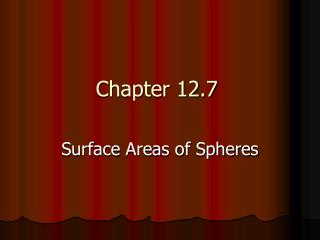Download PresentationChapter 12.7

# Chapter 12.7

Download Presentation## Chapter 12.7

- - - - - - - - - - - - - - - - - - - - - - - - - - - E N D - - - - - - - - - - - - - - - - - - - - - - - - - - -
##### Presentation Transcript

1. Chapter 12.7 Surface Areas of Spheres

2. Objectives • Recognize and define basic properties of spheres • Find surface areas of spheres

3. Parts of a Sphere G Sphere D • Point D is the center of the sphere • AB is the diameter of sphere D • DC, DA, and DB and radii • FG and AB are chords • JH is a tangent to sphere D at point E F D B A C E H J

4. a circle a point - The intersection of a plane and a sphere can be or . - When a plane intersects a sphere so that it contains the center of the sphere, the intersection is called . (Note: A great circle has the same center as the sphere, and its radii are also radii of the sphere.) a great circle

5. A Circle A Point No Intersection

6. Center A great Circle

7. Each Great circle divides a sphere into two halves, each called a hemi- sphere.

8. Example 1: In the figure, C is the center of the sphere, and plane R intersects the sphere in circle X. If XC = 9 centimeters and CY = 30 centimeters, find XY. Triangle CXY is a right triangle. (Angle X = 90°) R 30 cm 9 cm XY² + XC² = YC² Pythagorean Theorem XY² + 9² = 30² Plug in numbers XY² + 81 = 900 Square Numbers XY² = 900 – 81 Subtract 81 from both sides XY² = 819 900 – 81 = 819 XY = √819 Find the square root of 819 XY ≈ 28.6 cm Punch it in the calculator…and you get the approximate answer

9. Area of a Sphere If a sphere has a surface area of A square units and a radius of r units, then A = 4πr². (A great circle’s area is πr²)

10. Example 2: Find the surface area of the sphere given the area of the great circle. We know that the surface area of a sphere is four times the area of the great circle. A = 4πr² Surface Area of a sphere ≈ 4(603.3) πr² ≈ 603.3 ≈ 2413.2 Multiply The surface area of this sphere is ≈ 2413.2 in.² G ≈ 603.3 in.²

11. 8.4 cm Find the surface area of the hemisphere. A hemisphere is half of a sphere. To find the surface area, find half of the surface area of the sphere and add the area of the great circle. Surface area = ½ (4πr²) + πr² Surface area of a hemisphere = ½ [4π(8.4)²] + π(8.4)² Substitution ≈ 664.7 Use a calculator The surface area of the hemisphere is approximately 664.7cm²

12. Example 3: Find the surface area of a baseball given the circumference of 9 inches to determine how much leather is needed to cover the ball. First find the radius of the ball. C = 2πrCircumference of a circle 9 = 2πr Substitution 9/2π = r Division 1.4 ≈ r Use a calculator

13. Next, find the surface area. A = 4πr² Surface area of a sphere ≈ 4π(1.4)² Substitution ≈ 25.8 Use a calculator The surface area of the baseball is approximately 25.8 inches²

14. Assignment Pre-AP Geometry Page 674 # 10-29, #34 and #36